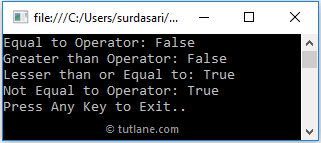# C# Relational Operators with Examples

In c#, Relational Operators are useful to check the relation between two operands like we can determine whether two operand values equal or not, etc., based on our requirements.

Generally, the c# relational operators will return true only when the defined operands relationship becomes true. Otherwise, it will return false.

For example, we have integer variables a = 10, b = 20. If we apply a relational operator >= (a >= b), we will get the result false because the variable “a” contains a value that is less than variable b.

The following table lists the different types of operators available in c# relational operators.

OperatorNameDescriptionExample (a = 6, b = 3)
== Equal to It compares two operands, and it returns true if both are the same. a == b (false)
> Greater than It compares whether the left operand greater than the right operand or not and returns true if it is satisfied. a > b (true)
< Less than It compares whether the left operand less than the right operand or not and returns true if it is satisfied. a < b (false)
>= Greater than or Equal to It compares whether the left operand greater than or equal to the right operand or not and returns true if it is satisfied. a >= b (true)
<= Less than or Equal to It compares whether the left operand less than or equal to the right operand or not and returns true if it is satisfied. a <= b (false)
!= Not Equal to It checks whether two operand values equal or not and return true if values are not equal. a != b (true)

## C# Relational Operators Example

Following is the example of using the Relational Operators in c# programming language.

using System;

namespace Tutlane
{
class Program
{
static void Main(string[] args)
{
bool result;
int x = 10, y = 20;
result = (x == y);
Console.WriteLine("Equal to Operator: " + result);
result = (x > y);
Console.WriteLine("Greater than Operator: " + result);
result = (x <= y);
Console.WriteLine("Lesser than or Equal to: "+ result);
result = (x != y);
Console.WriteLine("Not Equal to Operator: " + result);
Console.WriteLine("Press Enter Key to Exit..");
}
}
}

If you observe the above code, we used different Relational operators (<, >, ==) to perform required operations on defined operands.

## Output of C# Relational Operators Example

When we execute the above c# program, we will get the result as shown below.This is how we can use the relational operators in the c# programming language to check the relationship between defined operands based on our requirements.